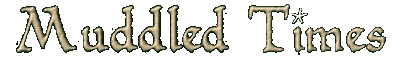Issue: Issue 9, April 2001 Section: Quizzes Author: Cordelia

# Know Thy Neighbour!!

How well do you know the other personae who populate The Land of Mud2? Here is the second part to the three part quiz that tests your knowledge of the names of personae known to frequent this world we call MUD2. Collect your answers together then send them to karya@muddled-times.com. The three people who obtain the highest scores will win the prize of 1000 points each to a non-mage persona, so get cracking! At the end of the series of quizzes there will be a cumulative prize also.

## The Word Search Round

This timeless classic is usually reserved for paper only, but using my ingenious and complicated system, you can indeed complete a word search on a computer! There are 20 persona names to find. When you have found a name, find the alphanumeric code for the first letter, put a dash, then the alphanumeric code for the last letter of the name. Confused? Good. Here’s an example: if someone was called Pose, the code for their name would be C1-F1 (if you’re looking, it’s on the left of the top line). Try and find all twenty names, and write the names of the codes down alphabetically, starting with any that start with the letter A, then move on to ones starting with the letter B, etc. Try and find them all, each one is worth one point. If you find a name which isn’t on my list, but by some freak accident it’s there, then you will get no bonus points, so don’t even try and create a persona just to get an extra point!

 A B C D E F G H I J K L M N O 1 P R P O S E C N B E R A Z G H 2 T U A D N L E S T A T F V R T 3 Q M R S O F T I O W C Q A X E 4 O B B I W Y B X M G E D Y H B 5 U R E P A O C B D W E V U Y A 6 Q A G N C B L P P Z J C H E Z 7 O R F A Y H K F O M K M F L I 8 R G J Z N M D A T L P I U X L 9 S Z O Q C D L D Z H E L U E E 10 V N A M T N A X S A G D X L N 11 G Y T N Y T M L L N R I R Y A 12 O E E R I C R K F Y K G N A M 13 S D I O K N A M M A N I A C E 14 W Z H F J X Y U J V S L K T H 15 J I U O L A Z A B A L A W Z T

See here for the first part "The Crytic Round" of this three-part quiz.
See here for the second part "The Anagram Round" of this three-part quiz.